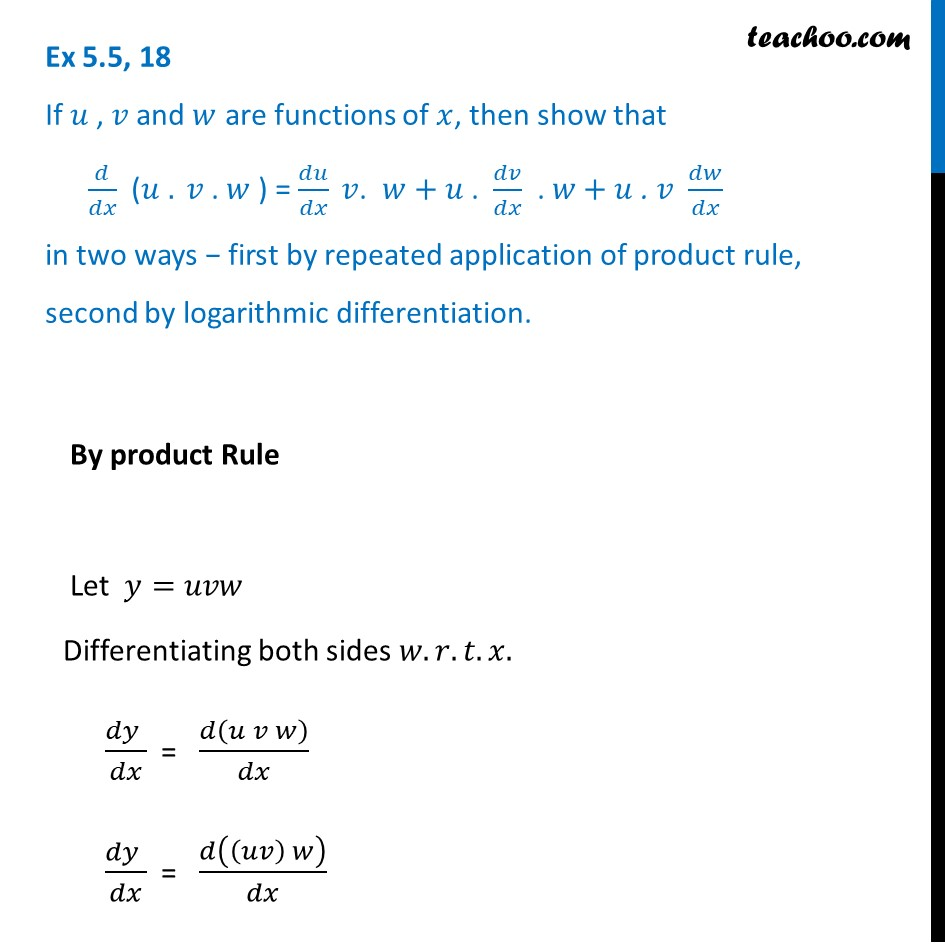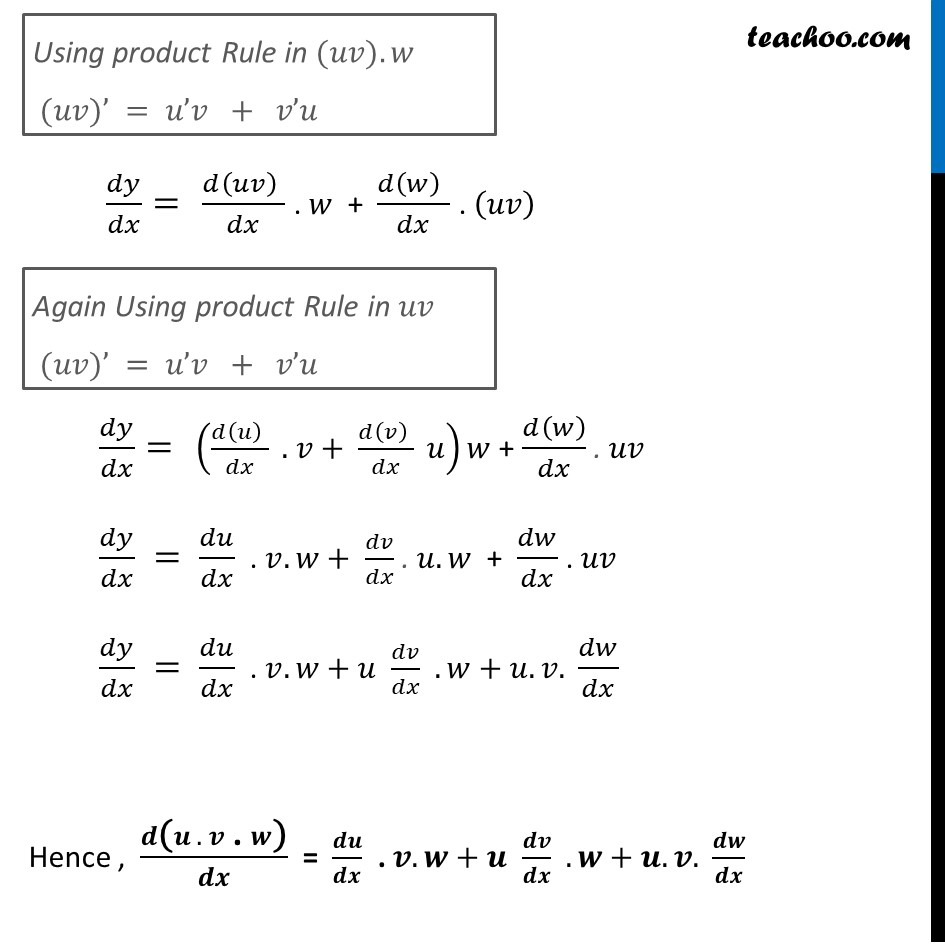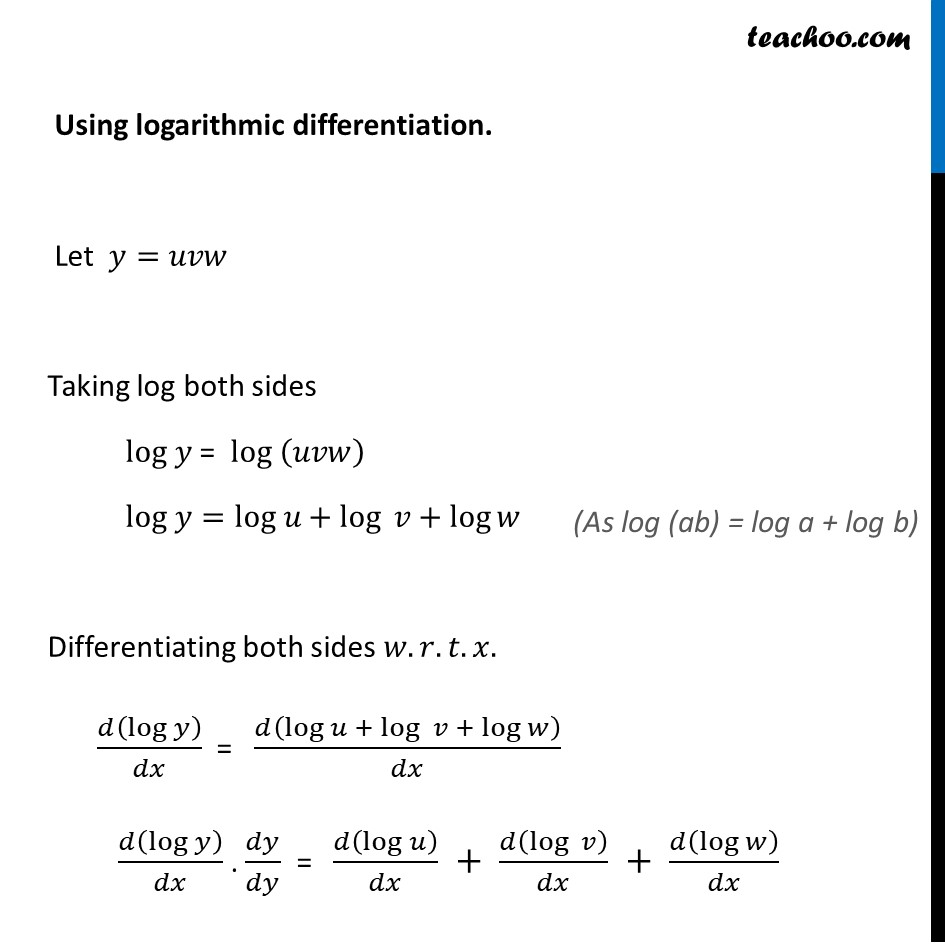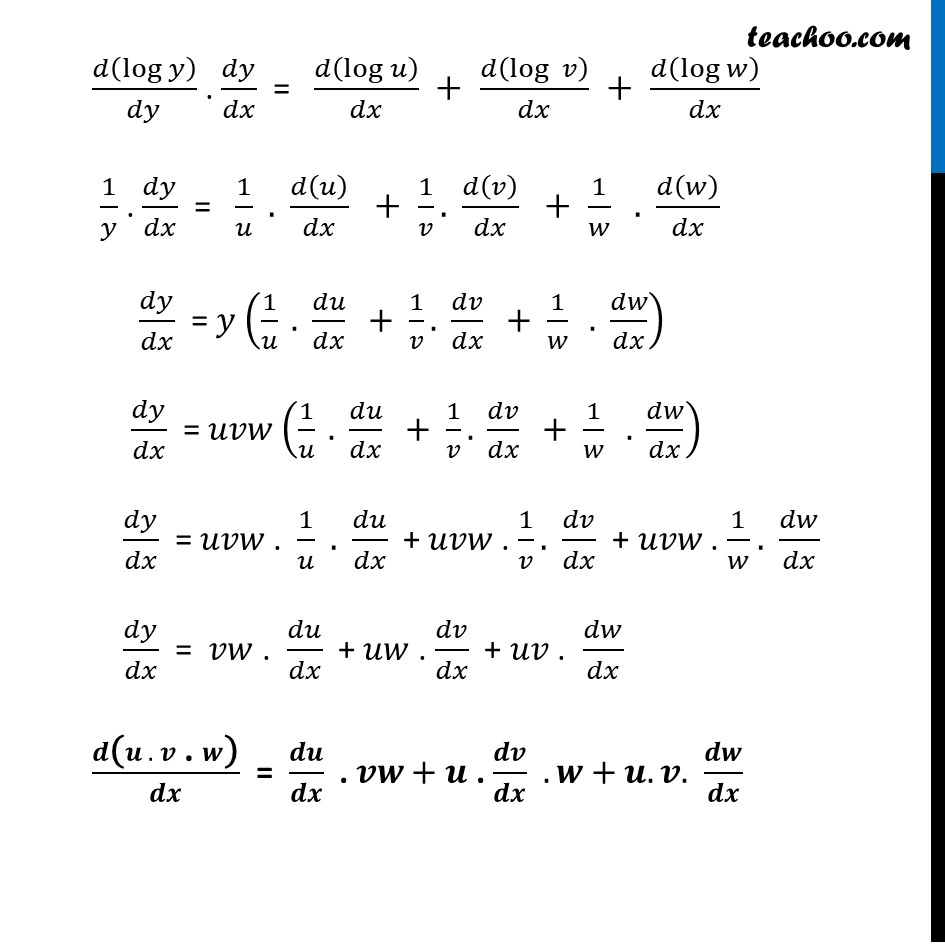Ex 5.5

Chapter 5 Class 12 Continuity and Differentiability
Serial order wiseGet live Maths 1-on-1 Classs - Class 6 to 12

### Transcript

Ex 5.5, 18 If 𝑢 , 𝑣 and 𝑤 are functions of 𝑥, then show that 𝑑/𝑑𝑥 (𝑢 . 𝑣 . 𝑤 ) = 𝑑𝑢/𝑑𝑥 𝑣. 𝑤+𝑢 . 𝑑𝑣/𝑑𝑥 . 𝑤+𝑢 . 𝑣 𝑑𝑤/𝑑𝑥 in two ways − first by repeated application of product rule, second by logarithmic differentiation. By product Rule Let 𝑦=𝑢𝑣𝑤 Differentiating both sides 𝑤.𝑟.𝑡.𝑥. (𝑑𝑦 )/𝑑𝑥 = 𝑑(𝑢 𝑣 𝑤)/𝑑𝑥 (𝑑𝑦 )/𝑑𝑥 = 𝑑((𝑢𝑣) 𝑤)/𝑑𝑥 Using product Rule in (𝑢𝑣). 𝑤 (𝑢𝑣)’ = 𝑢’𝑣 + 𝑣’𝑢 𝑑𝑦/𝑑𝑥= 𝑑(𝑢𝑣)" " /𝑑𝑥 . 𝑤 + 𝑑(𝑤)" " /𝑑𝑥 . (𝑢𝑣) 𝑑𝑦/𝑑𝑥= (𝑑(𝑢)" " /𝑑𝑥 " . " 𝑣+ 𝑑(𝑣)" " /𝑑𝑥 𝑢)𝑤 + 𝑑(𝑤)/𝑑𝑥 . 𝑢𝑣 𝑑𝑦/𝑑𝑥 = 𝑑𝑢/𝑑𝑥 . 𝑣.𝑤+ 𝑑𝑣/𝑑𝑥 . 𝑢.𝑤 + 𝑑𝑤/𝑑𝑥 . 𝑢𝑣 𝑑𝑦/𝑑𝑥 = 𝑑𝑢/𝑑𝑥 . 𝑣.𝑤+𝑢 𝑑𝑣/𝑑𝑥 .𝑤+𝑢.𝑣. 𝑑𝑤/𝑑𝑥 Hence , (𝒅"(" 𝒖 . 𝒗" . " 𝒘")" )/𝒅𝒙 = 𝒅𝒖/𝒅𝒙 . 𝒗.𝒘+𝒖 𝒅𝒗/𝒅𝒙 .𝒘+𝒖.𝒗. 𝒅𝒘/𝒅𝒙 Again Using product Rule in 𝑢𝑣 (𝑢𝑣)’ = 𝑢’𝑣 + 𝑣’𝑢 Using logarithmic differentiation. Let 𝑦=𝑢𝑣𝑤 Taking log both sides log 𝑦 = log (𝑢𝑣𝑤) log 𝑦=log 𝑢+〖log 〗⁡𝑣+log⁡𝑤 Differentiating both sides 𝑤.𝑟.𝑡.𝑥. 𝑑(log⁡𝑦 )/𝑑𝑥 = 𝑑(log 𝑢 + 〖log 〗⁡𝑣 + log⁡𝑤 )/𝑑𝑥 𝑑(log⁡𝑦 )/𝑑𝑥 . 𝑑𝑦/𝑑𝑦 = 𝑑(log 𝑢)/𝑑𝑥 + 𝑑(〖log 〗⁡𝑣 )/𝑑𝑥 + 𝑑(log⁡𝑤 )/𝑑𝑥 (As log (ab) = log a + log b) 𝑑(log⁡𝑦 )/𝑑𝑦 . 𝑑𝑦/𝑑𝑥 = 𝑑(log 𝑢)/𝑑𝑥 + 𝑑(〖log 〗⁡𝑣 )/𝑑𝑥 + 𝑑(log⁡𝑤 )/𝑑𝑥 1/𝑦 . 𝑑𝑦/𝑑𝑥 = 1/𝑢 . 𝑑(𝑢)/𝑑𝑥 + 1/𝑣. 𝑑(𝑣)/𝑑𝑥 + 1/𝑤 . 𝑑(𝑤)/𝑑𝑥 𝑑𝑦/𝑑𝑥 = 𝑦 (1/𝑢 . 𝑑𝑢/𝑑𝑥 + 1/𝑣. 𝑑𝑣/𝑑𝑥 + 1/𝑤 . 𝑑𝑤/𝑑𝑥) 𝑑𝑦/𝑑𝑥 = 𝑢𝑣𝑤 (1/𝑢 . 𝑑𝑢/𝑑𝑥 + 1/𝑣. 𝑑𝑣/𝑑𝑥 + 1/𝑤 . 𝑑𝑤/𝑑𝑥) 𝑑𝑦/𝑑𝑥 = 𝑢𝑣𝑤 . 1/𝑢 . 𝑑𝑢/𝑑𝑥 + 𝑢𝑣𝑤 . 1/𝑣. 𝑑𝑣/𝑑𝑥 + 𝑢𝑣𝑤 . 1/𝑤. 𝑑𝑤/𝑑𝑥 𝑑𝑦/𝑑𝑥 = 𝑣𝑤 . 𝑑𝑢/𝑑𝑥 + 𝑢𝑤 . 𝑑𝑣/𝑑𝑥 + 𝑢𝑣 . 𝑑𝑤/𝑑𝑥 (𝒅"(" 𝒖 . 𝒗" . " 𝒘")" )/𝒅𝒙 = 𝒅𝒖/𝒅𝒙 . 𝒗𝒘+𝒖 . 𝒅𝒗/𝒅𝒙 .𝒘+𝒖.𝒗. 𝒅𝒘/𝒅𝒙